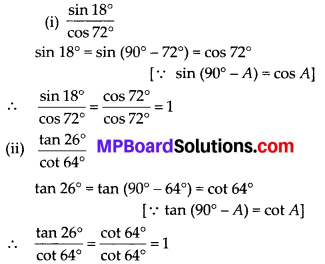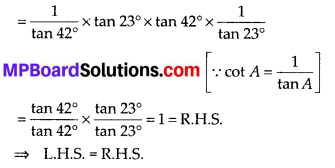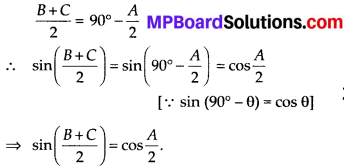# MP Board Class 10th Maths Solutions Chapter 8 Introduction to Trigonometry Ex 8.3

In this article, we will share MP Board Class 10th Maths Book Solutions Chapter 8 Introduction to Trigonometry Ex 8.3 Pdf, These solutions are solved subject experts from the latest edition books.

## MP Board Class 10th Maths Solutions Chapter 8 Introduction to Trigonometry Ex 8.3

Question 1.
Evaluate:
(i) $$\frac{\sin 18^{\circ}}{\cos 72^{\circ}}$$
(ii) $$\frac{\tan 26^{\circ}}{\cot 64^{\circ}}$$
(iii) cos48° – sin42°
(iv) cosec31°- sec59°
Solution:(iii) cos 48° – sin 42°
cos 48° = cos (90° – 42°) = sin 42°
[∵ cos (90° – A) = sin A]
∴ cos 48° – sin 42° = sin 42° – sin 42° = 0
(iv) cosec 31° – sec 59°
cosec 31° = cosec (90° – 59°) = sec 59° [ ∵ cosec (90° – A) = sec A]
∴ cosec 31° – sec 59° = sec 59° – sec 59° = 0

Question 2.
Show that:
(i) tan 48° tan 23° tan 42° tan 67° = 1
(ii) cos 38° cos 52° – sin 38° sin 52° = 0
Solution;
(i) L.H.S. = tan 48° tan 23° tan 42° tan 67°
= tan (90° – 42°) tan 23° tan 42° tan (90° – 23°)
= cot 42° tan 23° tan 42° cot 23° [ ∵ tan (90° – A) = cot A](ii) L.H.S. = cos 38° cos 52° – sin 38° sin 52°
= cos 38° cos (90° – 38°) – sin38° sin(90° – 38°)
= cos 38° sin 38° – sin 38° cos 38° [ ∵ sin(90° – A) = cosA and cos(90° – A)= sinA]
= 0 = R.H.S.
⇒ L.H.S. = R.H.S.Question 3.
If tan 2A = cot (A – 18°), where 2A is an acute angle, find the value of A.
Solution:
tan 2A = cot(A – 18°)
cot(90 – 2A) = cot (A – 18)
90 – 2A = A – 18
90 + 18 = A + 2A
3A= 108
A = $$\frac {108}{3}$$
A = 36°.

Question 4.
If tan A = cotB, prove that A + B = 90°.
Solution:
tan A = cot B and cot B = tan (90° – B) [∵ tan (90° – θ) = cot θ]
∴ A = 90° – B ⇒ A + B = 90°

Question 5.
If sec 4A = cosec (A – 20°), where 4A is an acute angle, find the value of A.
Solution:
sec 4A= cosec (A – 20)
cosec (90 – 4A) = cosec (A – 20)
90 – 4A = A – 20
90 + 20 =A + 4A
110 = 5A
A = $$\frac{110}{5}$$
A = 22°.Question 6.
If A, 6 and C are interior angles of a triangle ABC, then show that $$\sin \left(\frac{B+C}{2}\right)=\cos \frac{A}{2}$$
Solution:
Since, sum of the angles of ∆ABC is 180° i.e.,
A + B + C = 180°
∴ B + C = 180° – A
Dividing both sides by 2, we getQuestion 7.
Express sin 67° + cos 75° in terms of trigonometric ratios of angles between 0° and 45°.
Solution:
sin 67° + cos 75° = sin (90 – 23) + cos (90 – 15)
[sin(90 – θ) = cosθ
cos(90 – θ) = sinθ]
= cos 23 + sin 15